## CGAL::monotone_matrix_searchadvanced### Definition

The function monotone_matrix_search computes the maxima for all rows of a totally monotone matrix.

More precisely, monotony for matrices is defined as follows.

Let K be a totally ordered set,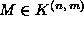a matrix over K and for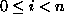: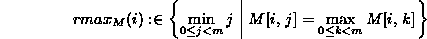the (leftmost) column containing the maximum entry in row i. M is called monotone, iff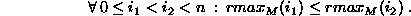M is totally monotone, iff all of its submatrices are monotone (or equivalently: iff all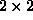submatrices are monotone).

#include <CGAL/monotone_matrix_search.h>

template < class Matrix, class RandomAccessIC, class Compare_strictly >
void
 monotone_matrix_search ( Matrix m, RandomAccessIC t, Compare_strictly compare_strictly = less< Matrix::Value >())

computes the maximum (as specified by compare_strictly) entry for each row of m and writes the corresponding column to t, i.e. t[i] is set to the index of the column containing the maximum element in row i. The maximum mr of a row r is the leftmost element for which compare_strictly(mr,x) is false for all elements x in r.

Precondition

t points to a structure of size at least m.number_of_rows()

Requirement

1. Matrix is a model for MonotoneMatrixSearchTraits.
2. Value type of RandomAccessIC is int.
3. If compare_strictly is defined, it is an adaptable binary function: Matrix::Value  × Matrix::Value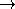bool describing a strict (non-reflexive) total ordering on Matrix::Value.

The implementation uses an algorithm by Aggarwal et al.[AKM+87]. The runtime is linear in the number of rows and columns of the matrix.advanced